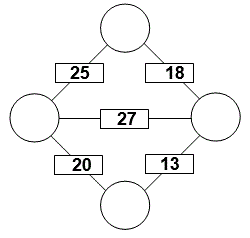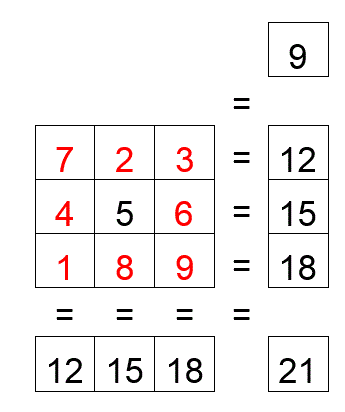Select Page

Each month, a new set of puzzles will be posted.  Come back next month for the solutions and a new set of puzzles, or subscribe to have them sent directly to you.

## MIND-Xpander puzzle

1. In a class of 78 students, 41 are taking French and 22 are taking German. Of the students taking French and German, 9 are taking both courses. How many students are not enrolled in either course?
2. You cash your paycheck and save half of it. With the rest, you pay a debt of \$20 and buy something for \$5. Then spending 1/5 of the remaining money, you have \$12 left. How much was your paycheck?

In the Arithmagon, the value in each box is the sum of the values in the circles on each side of it. Determine the value in each circle.## EQUATE+2 Puzzle

Each row, column & diagonal is an equation and you use the numbers 1 to 9 to complete the equations. Each number can be used only once. ‘Two’ numbers have been provided to get you started. Find the remaining eight numbers that satisfies all the resulting equations. Note: multiplication (x) & division (/) are performed before addition (+) and subtraction (-).## Feedback

There are more than one way of doing these puzzles and may well be more than one answer.  Please let me and others know what alternatives you find by commenting below.  We also welcome general comments on the subject and any feedback you'd like to give.

If you have a question that needs a response from me or you would like to contact me privately, please use the contact form.

## Get more puzzles!

If you've enjoyed doing the puzzles, consider ordering the books;

• Book One - 150+ of the best puzzles
• Book Two - 200+ with new originals and more of your favourites

Both in a handy pocket sized format.   Click here for full details.

## MIND-Xpander puzzle

Connect the 12 dots with 5 straight lines without lifting your pen or pencil off the paper or going through any dot twice. There are several solutions, depending on which outer dot you start with, but the pattern is still the same. Here’s our solution:## Can-U-Figure-It-Out puzzle

How many squares, of any size, are there in the following diagram? Solution:## EQUATE-Sums Puzzles

The missing numbers are between 1 and 9. Each number can be used only once. The numbers in each row add up to the totals on the right, and the columns adds up to the totals along the bottom. The sum of the two diagonals are also given. Solve the puzzle by finding all the missing numbers that satisfy all the sums as shown. Solutions: